## Example Questions

### Example Question #1741 : Act Math

Solve the following equation.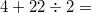Explanation:

This question is testing the order of operations. For the equation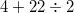, you must do the division problem first, then addition.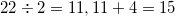.

### Example Question #2 : How To Add Integers

A functionis defined as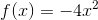. What is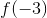?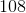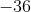Explanation:

To answer this question, we must substitute our value ofinto our equation forand follow our order of operations rules to solve for.

we first must substitute our known value into the equation. For this data: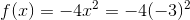We must now follow our order of operations to determine what we should do next. The order of operations goes as follows: parentheses, exponents, multiplication and division, and finally, addition and subtraction. So, for this equation, we must square thebecause solving for exponents comes before solving multiplication.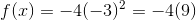Note that when you square a number, you are multiplying it by itself. When we multiply two negative numbers, their product is positive.

We then multiply our two integers together and solve for: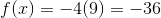Therefore, the answer to our question is.

### Example Question #1742 : Act Math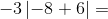Explanation:

To solve this question, we need to understand how absolute value works within an equation.

When numbers are within an absolute value sign (the numbers will be surrounded by), we need to use absolute value, or how far the number inside is away from zero. We must do any operations within the absolute value sign first. Therefore, the answer inside will always be positive, despite its sign, because you can't have negative distance from zero. So, for this data: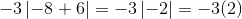We can then just multiply our two integers in order to get our answer: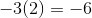Therefore, our answer is.

### Example Question #4 : How To Add Integers

If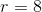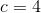, and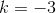, what does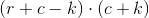equal?Explanation:

To answer this question, we must substitute our given values into an equation and solve, remembering our order of operations.

First, we must take our values for each variable and substitute them into the equation given. Therefore, since we are told that, and,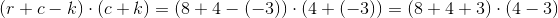Now that we have our values plugged in, we need to remember the order of operations in order to solve this problem. The order of operations goes as follows: parentheses, exponents, multiplication and division, and finally addition and subtraction. The first order of operations is to carry out any operations that are within parenthesis. Therefore, for this data: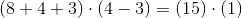We can now simply multiply these numbers together.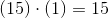Our answer is.

### Example Question #5 : How To Add Integers

Steven is bowling in a tournament and has the highest average after five games, with scores of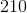,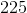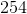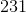, and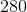. In order to maintain his average, what must be Steven's score on his sixth game?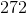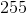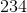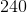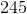Explanation:

To answer this question, we must calculate the average of Steven's five games. After this, to maintain his average, he needs to score the same in his sixth game as his average.

To calculate the average of a set of numbers, you add all the values together (or get the sum) and divide by the total number of values. So, for this data: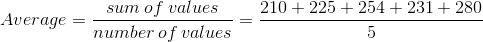We can then solve this fraction to get our average: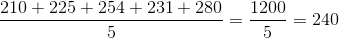Steven's average over the first five games is a score of. In order to maintain his average, he must match his average in game six. So, our answer is a score ofin game six.

### Example Question #1743 : Act Math

Stella’s Soda Shop has a 24 oz bag of ice that they purchased for $22.50. In each drink they sell, they use 2 oz of ice. If the soda shop sold 4 drinks, what is the value of the ice left in the bag? Possible Answers:$14.98

$7.52$16.86

$3.76$18.74

$14.98 Explanation: If the cost of the bag is$22.50 and the bag holds 24 oz, each ounce is worth 94 cents. If Stella sold 4 drinks with 2 oz of ice each, she has used 8 oz of ice. 8 oz of ice at 94 cents an ounce is valued at $7.52. If you subtract that from the total value of the bag of ice, that leaves$14.98 worth of ice.
If you answered $18.74, you only valued the ice in each drink at one ounce. If you answered$7.52, you found the value of ice in the drinks but not the value of the remainder of the bag.
If you answerd $3.76, you found the value of ice in the drinks if each drink only contained 1 ounce of ice. If you answered$16.86, you just forgot to subtract the cost of ice for all four drinks but rather only subtracted the cost of ice for three drinks.